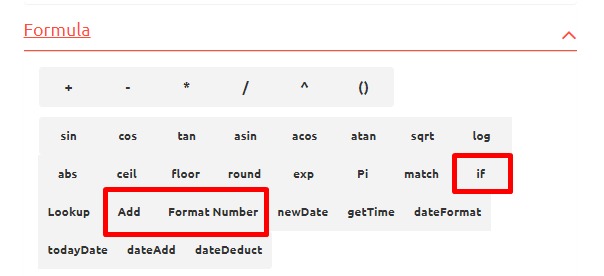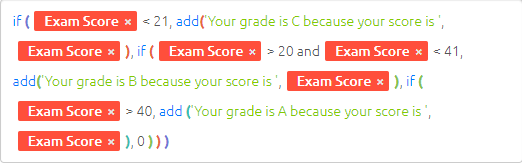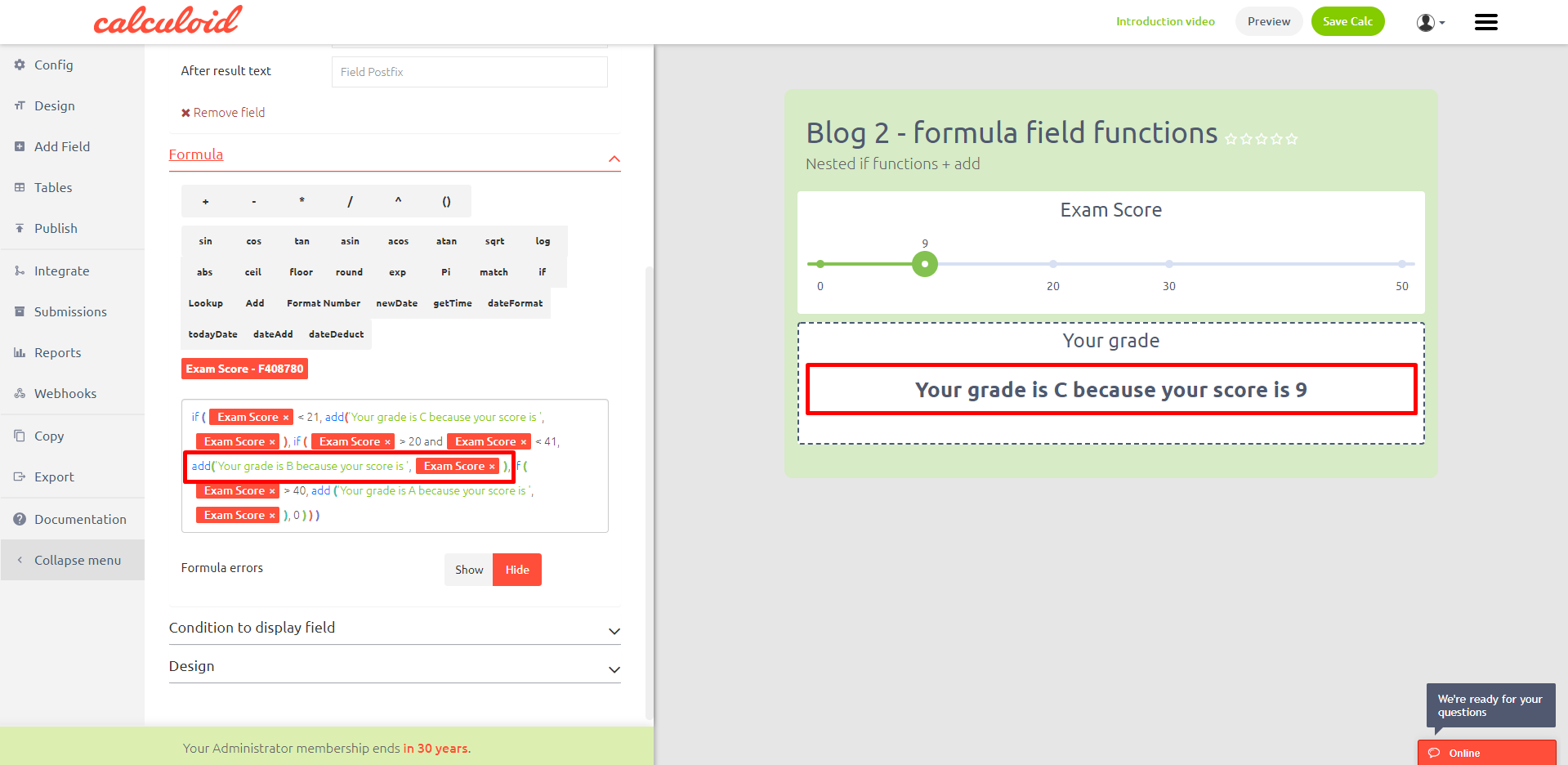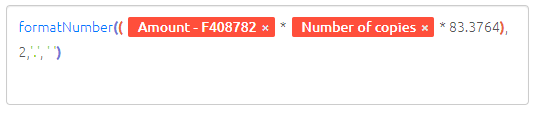Content

# Web calculators case studies #2 – Formula field functions

Functions in the formula field are strong features which allow creating of really smart web calculators. Furthermore, they help to format the results in the way you need.

Explore case studies and get inspired on how to use Formula field and functions to grow your business in Calculoid webinar recording.

Formula field in Calculoid was designed for math calculations. To give our users more possibilities we’ve added most used functions such as if, lookup, add ext. In our first blog, we showed how to use CSV tables with the lookup function. This blog is about nested if functions and two formatting functions: ‘add’ and ‘format-number’.## Case study 1 – Nested IF functions

Imagine creating a calculator with a lot of conditions. In such a case, you need to check all of them step by step and then present the results based on users choice. The best way to do so is nested if functions.Description of nested if function: if (condition, true, if (condition, true, if (condition, true, else))). Please note that you can put the next if function instead of true or else based on your needs.

The functions combined this way will check all the conditions determined step by step and present all the results based on users choice.

## Case study 2 – Add function

Add function is very important formatting function. It allows combining string (text) and integers (numbers).

You can create a message for each possible answer and combine it with the numerical results of your calculation as shown in the example calculator.## Case study 3 – Format number function

Format number is also a very popular formatting function. Mostly used in financial calculators. It allows formatting results of your calculation due to your standards. With these functions, you can set the number of decimal numbers, decimal delimiter and thousand delimiter.Description of format-number function: formatNumber(number, length of decimal,’decimal delimiter’, ‘thousand delimiter’).